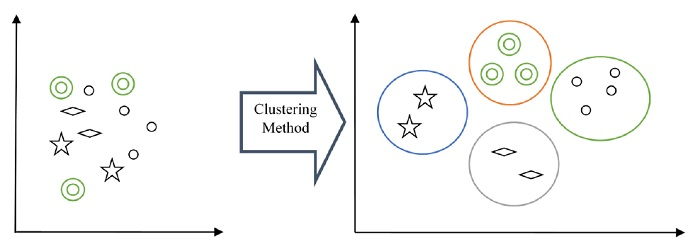# Which SciPy package is used to implement Clustering?

Clustering is one among the most useful unsupervised ML methods. It is used to find the relationship patterns and similarity among the input data samples. After finding these patterns, unsupervised algorithm clusters the data samples having similarities into groups as illustrated in the diagram below −Anomaly detection, image segmentation, medical imaging, social network analysis, and market segmentation are some common applications for clustering. K-means and Hierarchical are the two most common forms of clustering.

To implement clustering, SciPy provides us a clustering package (scipy.cluster) which further has two modules as given below −

• scipy.cluster.vq module − This SciPy module provides functions for k-means clustering and vector quantization. It also generates code books from k-means models by comparing them with centroids in a code book. The table below explains the routines, along with their description, consisting in scipy.cluster.vq module−

RoutineDescription
scipy.cluster.vq.whiten(obs, check_finite=True )This routine normalizes a group of observations on features.
scipy.cluster.vq.vq(obs, code_book,check_finite=True)This routine assigns codes from a codebook to observation.
scipy.cluster.vq.kmeans(obs, k_or_guess, iter=20, thresh=1e-05, check_finite=True)This routine performs k-means algorithms on a set of observation vectors forming kclusters.
scipy.cluster.vq.kmeans2(data,k,iter=10, thresh=1e-05, minit='random', missing='warn', check_finite=True)This routine classifies a set of observations into k-clusters by using the k-means algorithm.
• scipy.cluster.hierarchy module− As name suggested, this SciPy module provides functions for hierarchical clustering and its types such as agglomerative clustering. It has various routines which we can use to−

• Compute statistics on hierarchies

• Cut hierarchical clustering into the flat clustering.

• Implement agglomerative clustering.

• Visualize flat clustering.

• To check isomorphism of two flat cluster assignments.

• Plot the clusters.